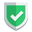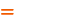我们相信：世界是美好的，你是我也是。平行空间的世界里面，不同版本的生活也在继续...

## `list`类型

``````import numpy as np
a =  [1, 2, 3]               # <class 'list'>
aa = [a, [4, 5, 6]]          # <class 'list'>
aa = [,[3,4,5,6]]         # <class 'list'>
# print(aa.shape)            # AttributeError: 'list' object has no attribute 'shape'``````

• `list`类型，可以表现为多重数组。
• `list`类型，相同层级的数据个数可以不同。
• `list`类型，没有`shape`属性。

## `nparray`类型

`nparray`从新定义的时候，必须保证每层元素数量一致。

``````a  = [[1, 2], [2, 3]]         # [[1, 2], [2, 3]] <class 'list'>
aa = np.array(a)              # <class 'numpy.ndarray'>``````

``aa = np.array([[1, 2], [3, 4]])  # <class 'numpy.ndarray'>``

``````aa = np.array([1,2],[3,4])    # TypeError: Field elements must be 2- or 3-tuples, got '3'
a  = [, [2, 3]]            # [, [2, 3]]    <class 'list'>
# aa = np.array(a)            # TypeError: unsupported operand type(s) for -: 'list' and 'int'``````

``````reating an ndarray from ragged nested sequences (which is a list-or-tuple of lists-or-tuples-or ndarrays with different lengths or shapes) is deprecated.
TypeError: Field elements must be 2- or 3-tuples, got '3'``````

``````a1 = np.random.randint(1, 3, size=(2, 3))    # <class 'numpy.ndarray'>
# [[2 2 2]
#  [2 1 2]]``````

``````a1 = np.random.randint(1, 3, size=(2, 3，4))    # <class 'numpy.ndarray'>
# [[[2 1 1 1]
#   [2 2 2 1]
#   [1 1 2 1]]
#  [[2 1 2 2]
#   [1 1 2 1]
#   [2 2 2 1]]]``````

## `nparray`类型的`shape`属性

``````a1 = np.array([[1, 2], [2, 3]])               # (2, 2)
a2 = np.random.randint(1, 3, size=(2, 3, 4))  # (2, 3, 4)
print(a1.shape,a2.shape)
exit()``````

## 访问元素

``````a = [[1, 2], [2, 3]]
a = 9
print(a) # [[9, 2], [2, 3]]

a1 = np.array([[1, 2], [2, 3]])
a1 = 9
print(a1)
# [[9 2]
#  [2 3]]``````

## nparray可以做加减法

``````import numpy as np
b1 = np.array([2, 3, 4])  #  ValueError: operands could not be broadcast together with shapes (3,) (2,)
b1 = np.array([[2, 3],[3,4]])
b2 = np.array([1, 1])
b3 = b1-b2
b4 = b1+b2
print(b3, b4)``````

``````[[1 2]
[2 3]]

[[3 4]
[4 5]]``````

## 综述

`python`中，`list``nparray`两者都可以是多重数组，都可以使用`[]`进行元素访问或者修改。

• 传统的多重数组就是`list`，每层元素数量可以不一致。
• 如果要求数量一致，就是`nparray`类型。个人理解着，`nparray`就是个带着框架模具萝卜坑的`array`

• `nparray`可以使用`.randomint()`初始化多层shape的数据。
• `nparray`从具体的数据初始化的话，记得满足同层元素数量相等的原则的前提下，还需要注意函数的唯一参数的最外侧由`[]`进行包裹，否则`list`类型会被识别成`tuple`，进而导致错误失败。如果本文对您有帮助，或者节约了您的时间，欢迎打赏瓶饮料，建立下友谊关系。本博客不欢迎：各种镜像采集行为。请尊重原创文章内容，转载请保留作者链接。【福利】 腾讯云最新爆款活动！1核2G云服务器首年50元！【源码】本文代码片段及相关软件，请点此获取更多信息【绝密】秘籍文章入口，仅传授于有缘之人python